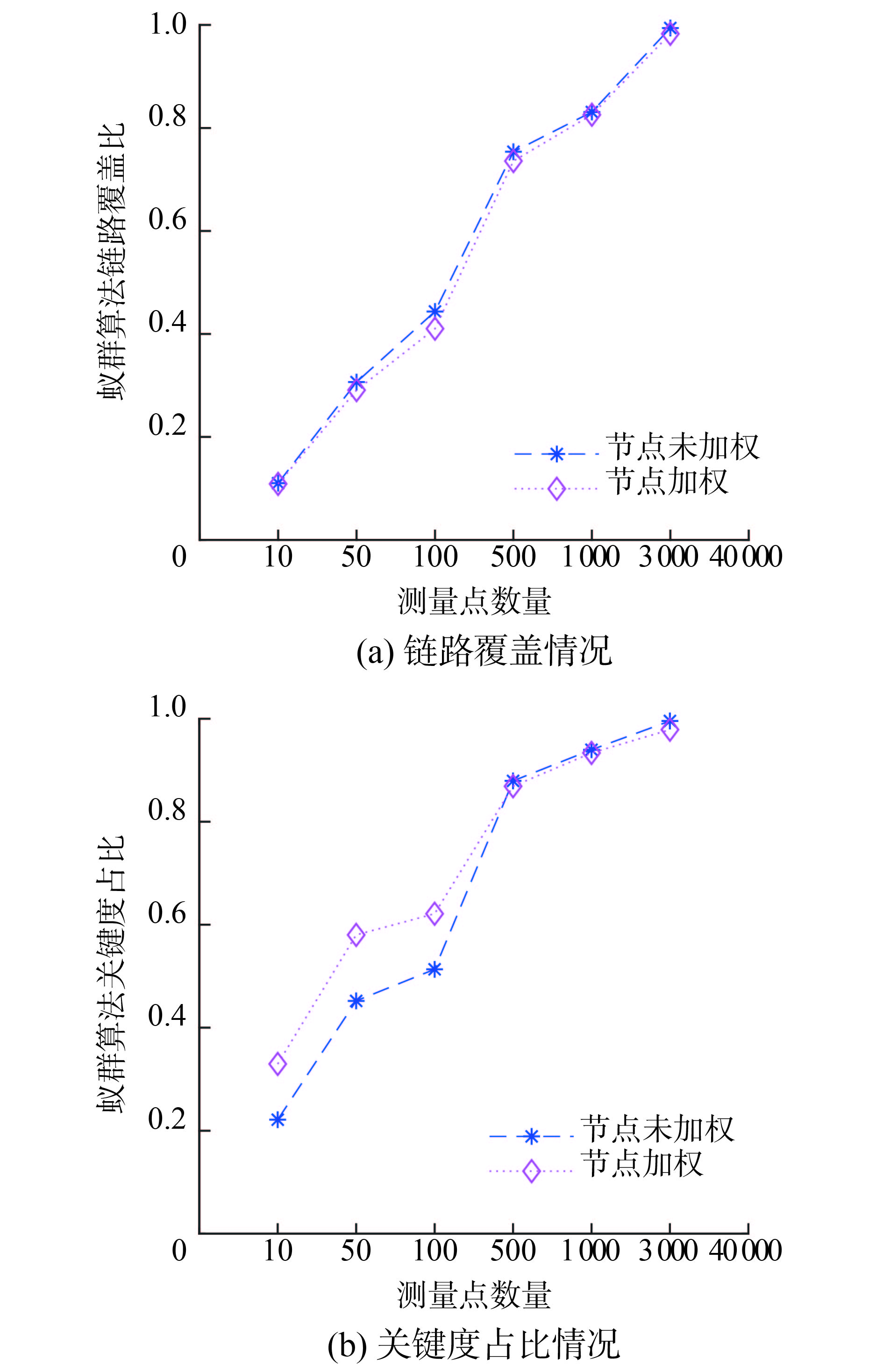﻿ 基于节点加权的网络流量测量点选择算法
«上一篇文章快速检索 高级检索

 应用科技2019, Vol. 46Issue (3): 86-92  DOI: 10.11991/yykj.2019030020

### 引用本文ZHAI Yujuan, LUO Hao, WU Zhigang, et al. Network traffic measurement nodes selection algorithm based on weighted nodes[J]. Applied Science and Technology, 2019, 46(3), 86-92. DOI: 10.11991/yykj.201903002.### 文章历史

Network traffic measurement nodes selection algorithm based on weighted nodes
ZHAI Yujuan, LUO Hao , WU Zhigang , ZHANG Shuzhuang
Institute of Network technology, Beijing University of Posts and Telecommunications, Beijing 100876, China
Abstract: In order to solve the problem that the existing algorithms cannot select the traffic measurement nodes according to the importance of different nodes to the network traffic transmission, this paper proposes a network traffic measurement nodes selection algorithm based on weighted nodes. It firstly assigns weight to nodes by node criticality, then uses correlation matrix approximation algorithm based on weighted nodes to calculate the initial solution, and finally, the algorithm is improved by pheromone initialization and expected heuristic information value calculation in the basic ant colony algorithm, forming the ant colony algorithm based on weighted nodes to calculate final solution of the problem. The experimental results show that the network traffic measurement nodes selection algorithm based on weighted nodes can prioritize the nodes with higher criticality on the premise of ensuring the link coverage.
Keywords: network measurement    measurement nodes    network traffic    ant colony algorithm    criticality    weighted nodes    approximation algorithm    network and information security

1 相关工作

1)节点度

2)介数

 ${{B}}\left( i \right) = \mathop \sum \nolimits_{s \ne i \ne t} \frac{{n_{st}^i}}{{{n_{st}}}}$

1）选择一个包含链路数量最多的节点，记做v1

2）在关联矩阵中删除v1对应的行以及该行中元素1所对应的列；

3）在剩下的关联矩阵中依次删除所有行元素之和不超过1的其他行以及这些行中元素1所在列，重复此步骤直到不能再删除新行为止；

4）重复以上步骤，直到关联矩阵中所有元素都被删除。

1)初始化

2)选择节点

 $p_i^k = \frac{{\tau _i^\alpha \eta _i^\beta }}{{ \displaystyle\sum \nolimits_{j \in {C_k}} \tau _j^\alpha \eta _j^\beta }}$ (1)

3)信息素更新

 ${\tau _i}\left( {t + 1} \right) = \left( {1 - \rho } \right){\tau _i}\left( t \right)$ (2)

 $\Delta {\tau _i} = \frac{Q}{{1 + \left| {\left| {{c_{{\rm{MVC}}}}} \right| + \left| {{g_{{\rm{MVC}}}}} \right|} \right|}}$ (3)

2 基于节点加权的网络流量测量点选择算法 2.1 问题描述及模型定义

 ${x_i} = \left\{ {\begin{array}{*{20}{c}} {{w_i},\;\;\;\;{v_i} \in S}\\ {0,\;\;\;\;\;\;{v_i} \notin S} \end{array}} \right.$ (4)

 ${\rm{min}}\mathop \sum \nolimits {x_i}$ (5)

2.2 算法实现

2.2.1 权重分配

 ${w_i} = \frac{1}{\sum \nolimits_{j \in N\left( i \right)} {c_j}}}$

2.2.2 初始解计算

 {a_{ij}} = \left\{ {\begin{aligned} {1,} & \;\;{{\text{节点}}{v_i}{\text{与}}{e_j}{\text{相关联}}}\\ {0,} & \;\;{{\text{其他}}} \end{aligned}} \right. (6)

 ${p_i} = \frac{{{w_i}}}{\sum \nolimits_{j = 1}^n {a_{ij}}}}$ (7)

2.2.3 基于节点加权的增强蚁群算法

1)信息素初始化

a)信息素初始值与节点权重成反比；

b)信息素初始值大小能反映权重的大小；

c)信息素初始值范围在[τminτmax]内。

 $\tau _{\min}' = \frac{1}{{{\tau _{\max}}}}$ (8)
 $\tau _{\max}' = \frac{1}{{{\tau _{\min}}}}$ (9)

 $\tau _i' = \tau _{\min}' + \left( {\tau _{\max}' - \tau _{\min}'} \right) \cdot \frac{{{w_i} - {w_{\min}}}}{{{w_{\max}} - {w_{\min}}}}$ (10)

 ${\tau _i} = \frac{1}{{\tau _i^{'}}}$ (11)

2)期望启发信息值的计算

 ${\eta _i} = \left| {{v_i}} \right| \cdot \mathop \sum \nolimits_{j \in {N_i}} {c_j}$ (12)

repeat

for蚁群中的每只蚂蚁

随机选择一个初始解集中的节点作为搜索的起始节点，并将其加入自己的覆盖集中

构造自己的候选点集合

while候选点集合不为空

每只蚂蚁根据公式（12）计算期望启发信息值

每只蚂蚁根据公式（1）计算状态转移概率，选择概率最大的节点加入最小弱顶点覆盖集

end while

end for

保留循环最优解

until最优解被找到或完成最大循环次数

3 实验评估

 $\left\{\begin{array}{l} {{x}} = \dfrac{{\displaystyle\sum \nolimits_{i \in R\left( i \right)} \left| {{v_i}} \right|}}{{\left| V \right|}}\\ {{y}} = \dfrac{\sum \nolimits_{i \in R\left( i \right)} {c_i}}}{\sum \nolimits_{{v_j} \in V} {c_j}}} \end{array}\right.$Download: 图 1 2种算法评估指标对比表 1 基本蚁群算法与节点加权的增强蚁群算法性能比较

4 结论

  AWDUCHE D, CHIU A, ELWALID A, et al. RFC 3272, Overview and principles of internet traffic engineering[S]. [S.l.]: RFC, 2002. (0)  SHU Zhaogang, WAN Jiafu, LIN Jiaxiang, et al. Traffic engineering in software-defined networking: measurement and management[J]. IEEE access, 2016, 4: 3246-3256. DOI:10.1109/ACCESS.2016.2582748 (0)  BREITBART Y, CHAN C Y, GAROFALAKIS M, et al. Efficiently monitoring bandwidth and latency in IP networks[C]//Proceedings IEEE INFOCOM 2001. Conference on Computer Communications. Twentieth Annual Joint Conference of the IEEE Computer and Communications Society. Anchorage, AK, USA, 2001: 933-942. (0)  刘湘辉, 殷建平, 唐乐乐, 等. 网络流量的有效测量方法分析[J]. 软件学报, 2003, 14(2): 300-304. (0)  蒋红艳, 林亚平, 黄生叶. 网络流量有效监测点的设置模型及求解算法研究[J]. 电子与信息学报, 2006, 28(4): 753-756. (0)  葛洪伟, 彭震宇, 岳海兵. 基于混合优化算法的网络流量有效测量点选择[J]. 计算机应用研究, 2009, 26(4): 1480-1483, 1486. DOI:10.3969/j.issn.1001-3695.2009.04.081 (0)  张荣, 金跃辉, 杨谈, 等. 分布式网络测量中测量节点的智能选择算法[J]. 计算机科学, 2015, 42(9): 70-77, 93. (0)  程光, 龚俭, 丁伟. 大规模互联网活动IP流分布研究[J]. 计算机科学, 2003, 30(4): 43-46. DOI:10.3969/j.issn.1002-137X.2003.04.012 (0)  胡满玉. 基于链接关系的有向加权复杂网络关键节点识别技术研究[D]. 南京: 南京理工大学, 2012. (0)  卢作维, 刘博元, 张沛, 等. 基于AS拓扑结构与网络服务分布的AS节点关键度分析[J]. 东南大学学报(自然科学版), 2017, 47(S1): 59-64. (0)  SHYU S J, YIN P Y, LIN B M T. An ant colony optimization algorithm for the minimum weight vertex cover problem[J]. Annals of operations research, 2004, 131(1/2/3/4): 283-304. (0)  王芳, 李美安, 段卫军. 基于动态自适应蚁群算法的云计算任务调度[J]. 计算机应用, 2013, 33(11): 3160-3162, 3196. (0)  GAO Lixin. On inferring autonomous system relationships in the Internet[J]. IEEE/ACM transactions on networking, 2001, 9(6): 733-745. DOI:10.1109/90.974527 (0)  LUCKIE M, HUFFAKER B, DHAMDHERE A, et al. AS relationships, customer cones, and validation[C]// Proceedings of 2013 Conference on Internet Measurement Conference. Barcelona, Spain, 2013. (0)